# Test: Exponents- 3

## 15 Questions MCQ Test Quantitative Reasoning for GMAT | Test: Exponents- 3

Description
Attempt Test: Exponents- 3 | 15 questions in 15 minutes | Mock test for GMAT preparation | Free important questions MCQ to study Quantitative Reasoning for GMAT for GMAT Exam | Download free PDF with solutions
QUESTION: 1

###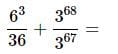Solution: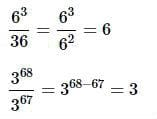Putting these together,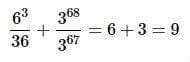QUESTION: 2

###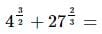Solution: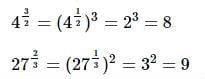Then putting them together,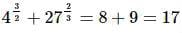QUESTION: 3

### Which of the following expressions is equivalent to this expression?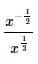You may assume that x>0.

Solution: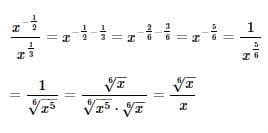QUESTION: 4

Simplify the following expression without a calculator: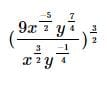Solution:

he easiest way to simplify is to work from the inside out. We should first get rid of the negatives in the exponents. Remember that variables with negative exponents are equal to the inverse of the expression with the opposite sign. For example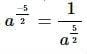So using this, we simplify: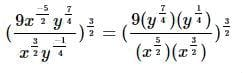Now when we multiply variables with exponents, to combine them, we add the exponents together. For example,  (a2)(a2)=a2+2=a4 Doing this to our expression we get it simplified to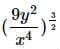The next step is taking the inside expression and exponentiating it. When taking an exponent of a variable with an exponent, we actually multiply the exponents. For example,  (a2)4=a(2∗4)=a8.
The other rule we must know that is an exponent of one half is the same as taking the square root. So for the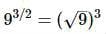So using these rules,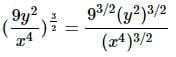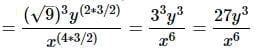QUESTION: 5

Rewrite as a single logarithmic expression:

3+log3x−2log3y

Solution:

First, write each expression as a base 3 logarithm:
3=log327 since 33=27
2log3y=log3y2
Rewrite the expression accordingly, and apply the logarithm sum and difference rules:
3+log3x−2log3y
=log327+log3x−log3y2

=log3(27⋅x)−log3y2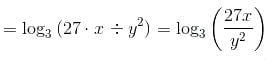QUESTION: 6

If 3p+3p+3p=3q, what is p in terms of q?

Solution:

We have 3p+3p+3p=3∗3p=3p+1=3q.

So p+1=q, and p=q−1.

QUESTION: 7

What are the last two digits, in order, of 6789 ?

Solution:

Inspect the first few powers of 6; a pattern emerges.

61=6

62=36

63=216

64=1,296

65=7,776

66=46,656

67=279,936

68=1,679,616

69=10,077,696

610=60,466,176

As you can see, the last two digits repeat in a cycle of 5.

789 divided by 5 yields a remainder of 4; the pattern that becomes apparent in the above list is that if the exponent divided by 5 yields a remainder of 4, then the power ends in the diigts 96.

QUESTION: 8

Which of the following expressions is equal to the expression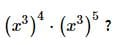Solution:

Use the properties of exponents as follows:

(x3)4⋅(x3)5

=x3⋅4 ⋅ x3⋅5

=x12⋅ x15

=x12+15

=x27

QUESTION: 9

Simplify: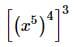Solution:

Apply the power of a power principle twice by multiplying exponents: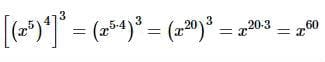QUESTION: 10

Divide:
(56x3+21x2−63x+77)÷7x2

Solution: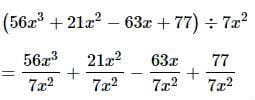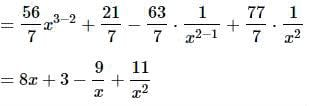QUESTION: 11

Solve for N: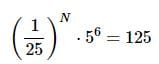Solution:

(1/25)⋅ 56=125

(5−2)⋅ 56=53

5−2⋅N ⋅ 56=53

5−2N + 6=53

−2N+6=3

−2N+6−6=3−6

−2N=−3

−2N÷(−2)=−3÷(−2)

N=3/2

QUESTION: 12

Which of the following is equal to log8+log5−log4 ?

Solution:

log8+log5−log4
=log(8⋅5)−log4
=log40−log4
=log(40÷4)
=log10=1

QUESTION: 13

Which of the following is equal to 4log3−2log6 ?

Solution:

4log3−2log6

=log(34)−log(62)

=log81−log36

=log81/36

=log9/4

=log 9− log 4

QUESTION: 14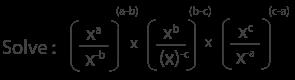Solution: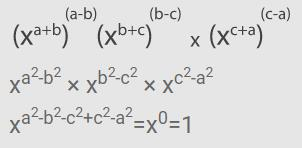QUESTION: 15

Which of the following is true about  log51,000 ?

Solution:

54=625;55=3,125

Therefore,

log625=4;log3,125=5

log5625<log51,000<log53,125

4<log51,000<5Use Code STAYHOME200 and get INR 200 additional OFF Use Coupon Code